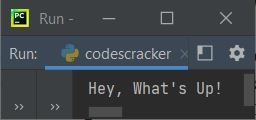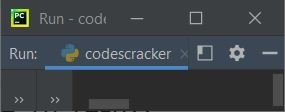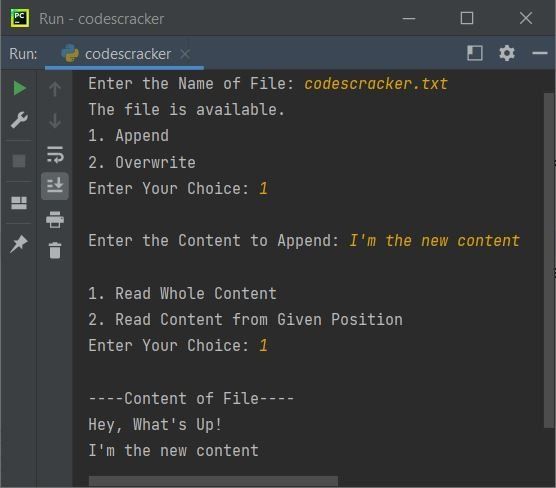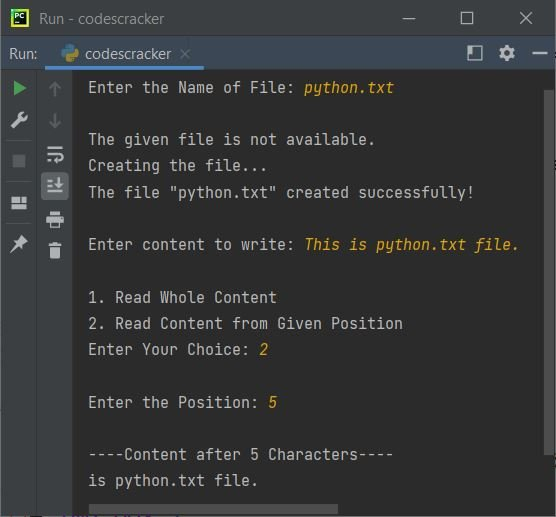# Python seek() Function

The seek() function in Python, used to set/return the position of the file pointer or handler, anywhere in the file. In other words, we can say that the function seek() is used to set or define the position of file handler, from where the read or write operation from/to the file has to perform.

## Python seek() Syntax

The syntax to use seek() function in a Python program is:

`fo.seek(offset, from_where)`

where fo indicates to the file object or file handler. The first parameter offset indicates to the value in number of bytes (characters) to start from.

The second parameter from_where indicates to the position from where the file object to start with. This parameter accepts following three values:

• 0 - to set the file handler at the beginning of the file
• 1 - to set the file handler to the current position
• 2 - to set the file handler at the end of the file

Note: File handler is like cursor. That is, where the cursor is in the file's content, from there the operation like reading, writing, updating performs.

Note: Providing only one parameter to seek() treats as the first parameter. The default value of from_where or second parameter of seek() is 0.

## Python seek() Example

Before using and demonstrating the seek() function in a Python program, let me first create a program, that creates a file say codescracker.txt if not available in the current directory. If available, then the content will be overwritten:

```fp = open("codescracker.txt", "w")
content = "Hey, What's Up!"
fp.write(content)
fp.close()

fp = open("codescracker.txt", "r")
print(content)```

If you execute this program, using any of the Python compiler say PyCharm, then the output produced by above program will be:If you open your current directory, then a file gets created or overwritten with Hey, What's Up!. Here is the snapshot of the current directory, including the opened newly created or overwritten file:Do you notice something in above program, the file object or handler fp gets closed after writing the content, so that to re-open the same file to read the file and print its content back on the screen. Let's create another program, that doesn't closes the file with its handler fp:

```fp = open("codescracker.txt", "w+")
content = "Hey, What's Up!"
fp.write(content)
print(content)```

Note: Must use w+ file opening mode, that allows read and write both the operation on the file.

Now the output produced by above program will be nothing like shown in the snapshot given below:Using the above program, because the file codescracker.txt already created using the first program, therefore the content Hey, What's Up! gets overwritten.

The output looks weird. This is because, while writing the content, the file handler fp goes to the end of the file after writing the last character, that was ! after Up. Therefore, we need to move the file handler fp at start of the file, so that the whole content gets read and printed on the output. Therefore let's modify the above program:

```fp = open("codescracker.txt", "w+")
content = "Hey, What's Up!"
fp.write(content)
fp.seek(0, 0)
print(content)```

Now this time, the output will be same as of first program's output. That is:In above program, the statement:

`fp.seek(0, 0)`

positioned the file handler fp to start from the beginning with 0th character.

Now let me create a program that will be the complete package to understand the way on how the seek() function works in Python. Do give your time to concentrate on the program very carefully to understand the complete thing with only one program. While understanding this program, may be, you can understand some more things including seek(). So let's begin the program:

```print("Enter the Name of File: ", end="")
filename = input()
try:
fo = open(filename, "r")
print("The file is available.")
print("1. Append")
print("2. Overwrite")
try:
choice = int(input())
if choice==1:
print("\nEnter the Content to Append: ", end="")
content = input()
fo.close()
fo = open(filename, "a+")
fo.write("\n")
fo.write(content)
print("2. Read Content from Given Position")
try:
choice = int(input())
if choice == 1:
fo.seek(0)
print("\n----Content of File----")
elif choice == 2:
print("\nEnter the Position: ", end="")
try:
position = int(input())
fo.seek(position)
print("\n----Content after", position, "Characters----")
except ValueError:
print("\nInvalid Input!")
else:
print("\nInvalid Choice!")
except ValueError:
print("\nInvalid Input!")
elif choice==2:
print("\nEnter the Content to Write (Overwrite): ", end="")
content = input()
fo.close()
fo = open(filename, "w+")
fo.write(content)
print("2. Read Content from Given Position")
try:
choice = int(input())
if choice == 1:
fo.seek(0)
print("\n----Content of File----")
elif choice == 2:
print("\nEnter the Position: ", end="")
try:
position = int(input())
fo.seek(position)
print("\n----Content after", position, "Characters----")
except ValueError:
print("\nInvalid Input!")
else:
print("\nInvalid Choice!")
except ValueError:
print("\nInvalid Input!")
except ValueError:
print("\nInvalid Input!")
except FileNotFoundError:
print("\nThe given file is not available.")
print("Creating the file...")
fo = open(filename, "w+")
print("The file \"", filename, "\" created successfully!", sep="")
print("\nEnter content to write: ", end="")
content = input()
fo.write(content)
print("2. Read Content from Given Position")
try:
choice = int(input())
if choice==1:
fo.seek(0)
print("\n----Content of File----")
elif choice==2:
print("\nEnter the Position: ", end="")
try:
position = int(input())
fo.seek(position)
print("\n----Content after", position, "Characters----")
except ValueError:
print("\nInvalid Input!")
else:
print("\nInvalid Choice!")
except ValueError:
print("\nInvalid Input!")
finally:
fo.close()```

Here is its sample run with user input codescracker.txt as name of file, 1 as choice, I'm the new content as content, 1 as choice:Note: The end= and sep=, both parameters are used to change the default behavior of print(). To learn in detail, refer to its separate tutorial.

Take a deep look at the above program and its sample run. You'll get to know everything about the seek() function. If there is only one parameter available to seek(), then it is considered as the first parameter, that is offset. Or only one parameter given to seek() means, the file position will be set from the beginning of the file, to the number of bytes provided by the value of the single parameter.

Here is another sample run with user input python.txt file (a non-existing file), This is python.txt file. as content to write in this file, 2 as choice to read the content of file from given position, and 5 as position from where we have to read the content as shown in the snapshot given below:As you can see, reading after first 5 characters from the file's content, that is This is python.txt file. means the first 5 characters that is:

1. T
2. h
3. i
4. s
5. (a space)

gets skipped. And all the characters after these 5 characters gets read as shown in the snapshot given below. The statement from above program:

`fo.seek(position)`

corresponds to the action of reading content after 5 characters. That is, when user enters 5 as position, means the code gets converted into:

`fo.seek(5)`

Therefore, using the following statement:

`print(fo.read())`

all the content of the file whose handler is fo, gets printed after the first five characters. That's it.

Python Online Test

« Previous Function Next Function »